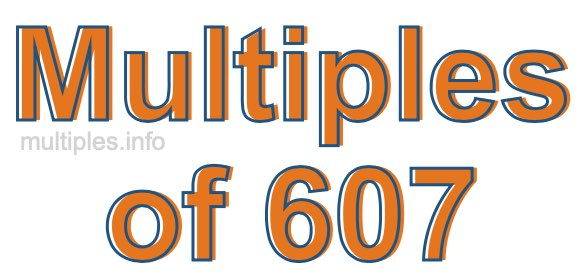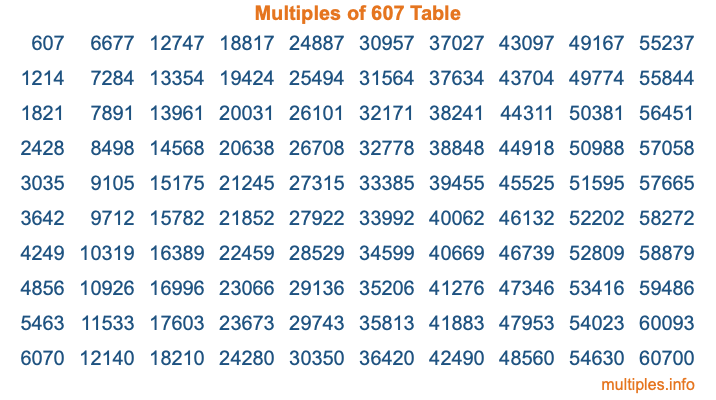Multiples of 607Welcome to the Multiples of 607 page. Here we will first teach you everything you will ever need to know about the multiples of 607, and then give you a study guide summary of everything we taught you to make sure you remember it all. Use this page to look up facts and learn information about the multiples of 607. This page will make you a multiples of six hundred seven expert!

Definition of Multiples of 607
Multiples of 607 are all the numbers that when divided by 607 equal an integer. Each of the multiples of 607 are called a multiple. A multiple of 607 is created by multiplying 607 by an integer.

Therefore, to create a list of multiples of 607, you start with 1 multiplied by 607, then 2 multiplied by 607, then 3 multiplied by 607, and so on for as long as you want. Thus, the list of the first five multiples of 607 is 607, 1214, 1821, 2428, and 3035. To see a larger list of multiples of 607, see the printable image of Multiples of 607 further down on this page. We also have a category where you can choose any nth multiple of 607.

Multiples of 607 Checker
The Multiples of 607 Checker below checks to see if any number of your choice is a multiple of 607. In other words, it checks to see if there is any number (integer) that when multiplied by 607 will equal your number. To do that, we divide your number by 607. If the the quotient is an integer, then your number is a multiple of 607.

Is  a multiple of 607?

Least Common Multiple of 607 and ...
A Least Common Multiple (LCM) is the lowest multiple that two or more numbers have in common. This is also called the smallest common multiple or lowest common multiple and is useful to know when you are adding our subtracting fractions. Enter one or more numbers below (607 is already entered) to find the LCM.

Check out our LCM Calculator if you need more details about the Least Common Multiple or if you need the LCM for different numbers for adding and subtraction fractions.

nth Multiple of 607
As we stated above, 607 is the first multiple of 607, 1214 is the second multiple of 607, 1821 is the third multiple of 607, and so on. Enter a number below to find the nth multiple of 607.

th multiple of 607

Multiples of 607 vs Factors of 607
607 is a multiple of 607 and a factor of 607, but that is where the similarities end. All postive multiples of 607 are 607 or greater than 607. All positive factors of 607 are 607 or less than 607.

Below is the beginning list of multiples of 607 and the factors of 607 so you can compare:

Multiples of 607: 607, 1214, 1821, 2428, 3035, etc.

Factors of 607: 1, 607

As you can see, the multiples of 607 are all the numbers that you can divide by 607 to get a whole number. The factors of 607, on the other hand, are all the whole numbers that you can multiply by another whole number to get 607.

It's also interesting to note that if a number (x) is a factor of 607, then 607 will also be a multiple of that number (x).

Multiples of 607 vs Divisors of 607
The divisors of 607 are all the integers that 607 can be divided by evenly. Below is a list of the divisors of 607.

Divisors of 607: 1, 607

The interesting thing to note here is that if you take any multiple of 607 and divide it by a divisor of 607, you will see that the quotient is an integer.

Multiples of 607 Table
Below is an image of the first 100 multiples of 607 in a table. The table is in chronological order, column by column. The first column has the first ten multiples of 607, the second column has the next ten multiples of 607, and so on.The Multiples of 607 Table is also referred to as the 607 Times Table or Times Table of 607. You are welcome to print out our table for your studies.

Negative Multiples of 607
Although not often discussed or needed in math, it is worth mentioning that you can make a list of negative multiples of 607 by multiplying 607 by -1, then by -2, then by -3, and so on, to get the following list of negative multiples of 607:

-607, -1214, -1821, -2428, -3035, etc.

Multiples of 607 Summary
Below is a summary of important Multiples of 607 facts that we have discussed on this page. To retain the knowledge on this page, we recommend that you read through the summary and explain to yourself or a study partner why they hold true.

There are an infinite number of multiples of 607.

A multiple of 607 divided by 607 will equal a whole number.

607 divided by a factor of 607 equals a divisor of 607.

The nth multiple of 607 is n times 607.

The largest factor of 607 is equal to the first positive multiple of 607.

607 is a multiple of every factor of 607.

607 is a multiple of 607.

A multiple of 607 divided by a divisor of 607 equals an integer.

607 divided by a divisor of 607 equals a factor of 607.

Any integer times 607 will equal a multiple of 607.

Multiples of a Number
Here you can get the multiples of another number, all with the same attention to detail as we did for multiples of 607 on this page.

Multiples of
Multiples of 608
Did you find our page about multiples of six hundred seven educational? Do you want more knowledge? Check out the multiples of the next number on our list!